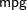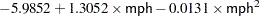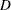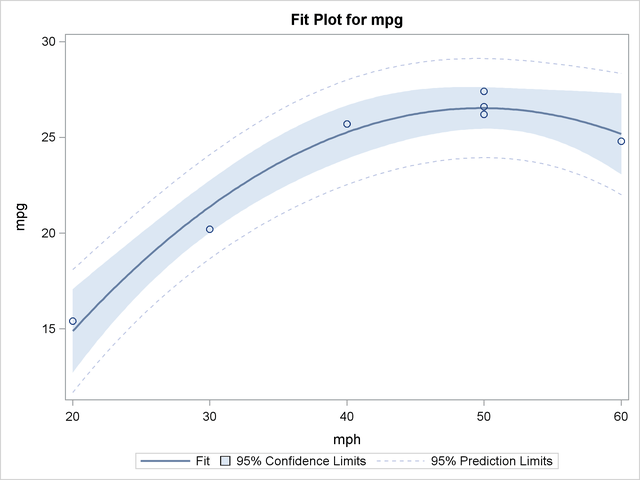## Example 41.2 Regression with Mileage Data

A car is tested for gas mileage at various speeds to determine at what speed the car achieves the highest gas mileage. A quadratic model is fit to the experimental data. The following statements produce Output 41.2.1 through Output 41.2.4.

```title 'Gasoline Mileage Experiment';
data mileage;
input mph mpg @@;
datalines;
20 15.4
30 20.2
40 25.7
50 26.2  50 26.6  50 27.4
55   .
60 24.8
;
```
```ods graphics on;
proc glm;
model mpg=mph mph*mph / p clm;
run;
ods graphics off;
```

Output 41.2.1 Standard Regression Analysis
 Gasoline Mileage Experiment

The GLM Procedure

 Number of Observations Read 8 7

 Gasoline Mileage Experiment

The GLM Procedure

Dependent Variable: mpg

Source DF Sum of Squares Mean Square F Value Pr > F
Model 2 111.8086183 55.9043091 77.96 0.0006
Error 4 2.8685246 0.7171311
Corrected Total 6 114.6771429

R-Square Coeff Var Root MSE mpg Mean
0.974986 3.564553 0.846836 23.75714

Source DF Type I SS Mean Square F Value Pr > F
mph 1 85.64464286 85.64464286 119.43 0.0004
mph*mph 1 26.16397541 26.16397541 36.48 0.0038

Source DF Type III SS Mean Square F Value Pr > F
mph 1 41.01171219 41.01171219 57.19 0.0016
mph*mph 1 26.16397541 26.16397541 36.48 0.0038

Parameter Estimate Standard Error t Value Pr > |t|
Intercept -5.985245902 3.18522249 -1.88 0.1334
mph 1.305245902 0.17259876 7.56 0.0016
mph*mph -0.013098361 0.00216852 -6.04 0.0038

The overallstatistic is significant. The tests of mph and mph*mph in the Type I sums of squares show that both the linear and quadratic terms in the regression model are significant. The model fits well, with anof 0.97. The table of parameter estimates indicates that the estimated regression equation isOutput 41.2.2 Results of Requesting the P and CLM Options
Observation   Observed Predicted Residual 95% Confidence Limits for Mean Predicted
Value
1   15.40000000 14.88032787 0.51967213 12.69701317 17.06364257
2   20.20000000 21.38360656 -1.18360656 20.01727192 22.74994119
3   25.70000000 25.26721311 0.43278689 23.87460041 26.65982582
4   26.20000000 26.53114754 -0.33114754 25.44573423 27.61656085
5   26.60000000 26.53114754 0.06885246 25.44573423 27.61656085
6   27.40000000 26.53114754 0.86885246 25.44573423 27.61656085
7 * . 26.18073770 . 24.88679308 27.47468233
8   24.80000000 25.17540984 -0.37540984 23.05954977 27.29126990

The P and CLM options in the MODEL statement produce the table shown in Output 41.2.2. For each observation, the observed, predicted, and residual values are shown. In addition, the 95% confidence limits for a mean predicted value are shown for each observation. Note that the observation with a missing value for mph is not used in the analysis, but predicted and confidence limit values are shown.

Output 41.2.3 Additional Results of Requesting the P and CLM Options
 Sum of Residuals -0 2.86852 -0 23.1811 -0.543766 2.94426

The last portion of the output listing, shown in Output 41.2.3, gives some additional information about the residuals. The Press statistic gives the sum of squares of predicted residual errors, as described in Chapter 4, Introduction to Regression Procedures. The First Order Autocorrelation and the Durbin-Watsonstatistic, which measures first-order autocorrelation, are also given.

Output 41.2.4 Plot of Mileage DataFinally, the ODS GRAPHICS ON command in the previous statements enables ODS Graphics, which in this case produces the plot shown in Output 41.2.4 of the actual and predicted values for the data, as well as a band representing the confidence limits for individual predictions. The quadratic relationship between mpg and mph is evident.